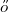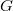﻿

### The Erd$\H{o}$s-Faber-Lovász Conjecture revisited

#### Abstract

The Erd$\H{o}$s-Faber-Lovász Conjecture, posed in 1972, states that if a graph$G$ is the union of$n$ cliques of order$n$ (referred to as defining$n$-cliques) such that two cliques can share at most one vertex, then the vertices of$G$ can be properly coloured using$n$ colours. Although still open after almost 50 years, it can be easily shown that the conjecture is true when every shared vertex belongs to exactly two defining$n$-cliques. We here provide a quick and easy algorithm to colour the vertices of$G$ in this case, and discuss connections with clique-decompositions and edge-colourings of graphs.

DOI Code: 10.1285/i15900932v41n2p1

Keywords: Erdös-Faber-Lovász Conjecture; chromatic number; clique-decomposition; edge-colouring

Full Text: PDF